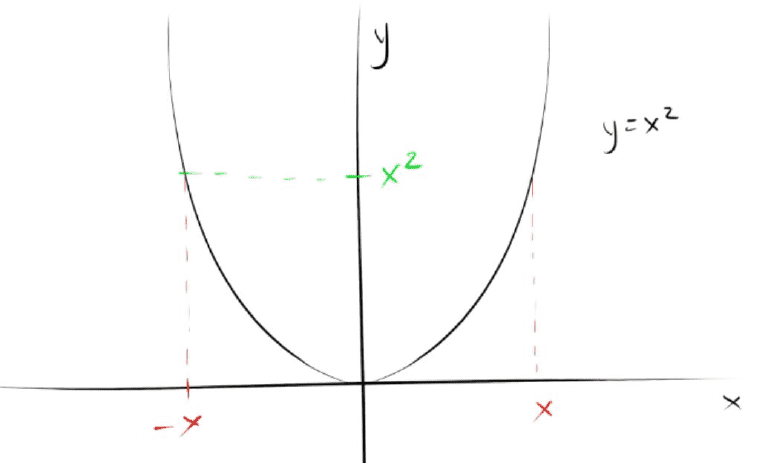Get 2 days of premium access
Class Notes (1,000,000)
US (430,000)
UC-Irvine (10,000)
MATH (1,000)
MATH 2A (400)
Lecture 1

# MATH 2A Lecture Notes - Lecture 1: Set Notation, PiecewisePremium

Department
Mathematics
Course Code
MATH 2A
Professor
Shuhao Cao
Lecture
1

This preview shows pages 1-2. to view the full 6 pages of the document.Math 2A Calculus 44095
Week 0 Lecture 1 Functions
Functions
  
o This symbol represents the set of all real numbers
o Written as letter R with a line cutting through it vertically
 
o Means that x is in the set R (R is a real number)
o This new symbol denotes that x belongs to (or is in, or an element of) R
Intervals
Open Interval
o (-3, 5) = this states that x is a value from -3 to 5;     
Closed Interval
o [-3, 5] = this states that x is a value from -3 to 5 including 3 and 5;  
 
Hybrids
o (-3, 5]
Means that     
o [-3, 5)
Means that     
Joining Intervals
o 
This union symbol indicates a joining
Such that      or     
Domain and Range
Input Output
o With being the function
o Input referring to the domain
o Output referring to the range
A function can be thought of as a processor
o (has both an input and an output)
Domain
o Is typically given the notation
o Refers to the set of all sensible inputs for the function given
o Example:
In this example, the domain cannot be negative, as it would yield an
impossible answer
So therefore,   

Only pages 1-2 are available for preview. Some parts have been intentionally blurred.

Subscribers OnlyThe second section of the above indicates the set notation
for a collection of numbers
Range
o Range   
o The collection of possible outcomes
Found by plugging in the domain to the function
Means that of in which has to be within the domain given
o  
Piecewise Function
   
 
 
Domain is in two parts, and if it doesn’t fall into the first category, it falls into the
other
Symmetry
Even functions are functions such that  
o These are symmetric with respect to the y-axis
o
Odd functions are functions such that 
o These are symmetric with respect to the origin
###### You're Reading a Preview

Unlock to view full version

Subscribers Only